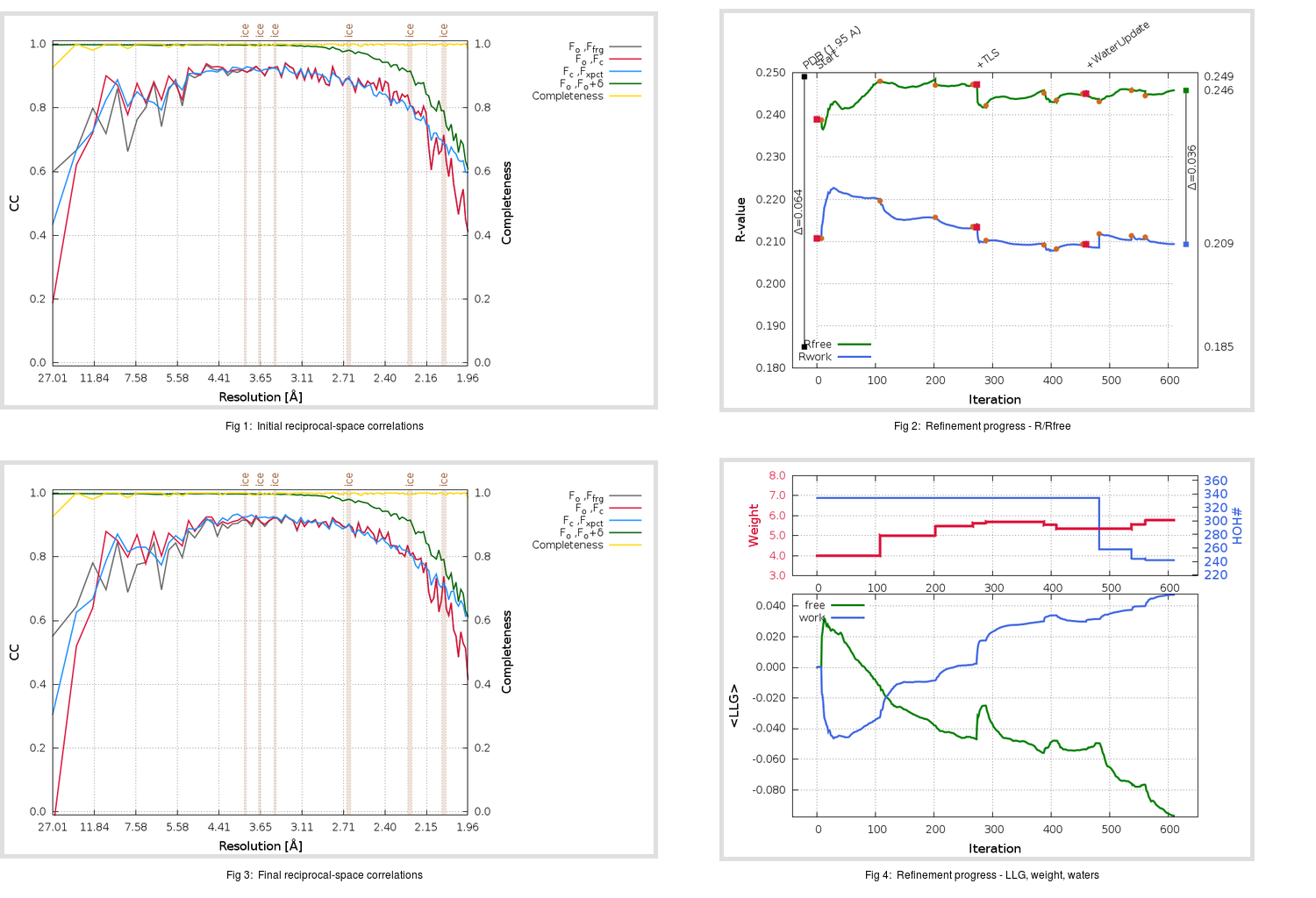Content:

```    Diffraction limits & principal axes of ellipsoid fitted to diffraction cut-off surface:
1.975         0.9278   0.0000   0.3731       0.997 a* + 0.074 c*
1.875         0.0000   1.0000   0.0000       b*
1.860        -0.3731   0.0000   0.9278      -0.696 a* + 0.718 c*
```

## Deposited

` `
 Date deposited Date data collection Resolution R, Rfree 20200303 20200226 1.95 0.1820 0.2490

Molprobity (CCP4 7.0 version) summary:

```Ramachandran outliers =   0.33 %
favored =  97.02 %
Rotamer outliers      =   2.27 %
C-beta deviations     =     0
Clashscore            =   5.69
RMS(bonds)            =   0.0135
RMS(angles)           =   1.86
MolProbity score      =   1.75
Resolution            =   1.95
R-work                =   0.1820
R-free                =   0.2490
```

```Number of waters      =   334

<B> (all atoms) =   44.05 ( sd =   14.24 ) for       2735 non-hydrogen atoms
<B>   (protein) =   42.79 ( sd =   12.36 ) for       2370 non-hydrogen atoms
<B>     (water) =   51.60 ( sd =   17.82 ) for        334 non-hydrogen atoms
<B>    (others) =   92.62 ( sd =   59.60 ) for         31 non-hydrogen atoms

B min/max       (all non-hydrogen atoms) =   24.01 /  179.18
B min/max   (protein non-hydrogen atoms) =   24.01 /  112.14
B min/max     (water non-hydrogen atoms) =   25.92 /  137.71
B min/max     (other non-hydrogen atoms) =   43.99 /  179.18
```

## BUSTER (re-)refinement

` `

Molprobity (CCP4 7.0 version) summary:

```Ramachandran outliers =   0.33 %
favored =  98.01 %
Rotamer outliers      =   1.52 %
C-beta deviations     =     0
Clashscore            =   2.74
RMS(bonds)            =   0.0119
RMS(angles)           =   1.56
MolProbity score      =   1.20
Resolution            =   1.95
R-work                =   0.2094
R-free                =   0.2459
```

```Number of waters      =   242

<B> (all atoms) =   42.77 ( sd =    9.33 ) for       2643 non-hydrogen atoms
<B>   (protein) =   42.44 ( sd =    8.39 ) for       2370 non-hydrogen atoms
<B>     (water) =   44.60 ( sd =   16.46 ) for        242 non-hydrogen atoms
<B>    (others) =   60.45 ( sd =   22.26 ) for         31 non-hydrogen atoms

B min/max       (all non-hydrogen atoms) =   22.44 /   91.51
B min/max   (protein non-hydrogen atoms) =   28.69 /   79.77
B min/max     (water non-hydrogen atoms) =   22.44 /   73.00
B min/max     (other non-hydrogen atoms) =   48.33 /   91.51
```

Refinement progression:Results:

` `
 File Remark 5R81_aB_refine.01_03_refine.pdb.gz exact refinement commands are in header 5R81_aB_refine.01_03_refine.mtz.gz including original deposited data and several re-refinement map coefficients 5R81_aB_refine.01_03_BUSTER_model.cif.gz including any non-standard compound restraints 5R81_aB_refine.01_03_BUSTER_refln.cif.gz